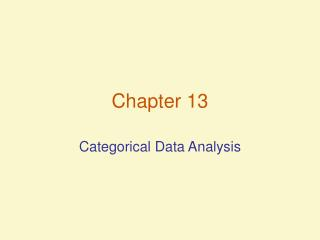Download PresentationChapter 13

# Chapter 13

Download Presentation## Chapter 13

- - - - - - - - - - - - - - - - - - - - - - - - - - - E N D - - - - - - - - - - - - - - - - - - - - - - - - - - -
##### Presentation Transcript

1. Chapter 13 Categorical Data Analysis

2. Categorical Data and the Multinomial Distribution • Properties of the Multinomial Experiment • Experiment has nidentical trials • There are k possible outcomes to each trial, called classes, categories or cells • Probabilities of the k outcomes remain constant from trial to trial • Trials are independent • Variables of interest are the cell counts, n1, n2…nk, the number of observations that fall into each of the k classes

3. Testing Category Probabilities: One-Way Table • In a multinomial experiment with categorical data from a single qualitative variable, we summarize data in a one-way table.

4. Testing Category Probabilities: One-Way Table • Hypothesis Testing for a One-Way Table • Based on the 2 statistic, which allows comparison between the observed distribution of counts and an expected distribution of counts across the k classes • Expected distribution = E(nk)=npk, where n is the total number of trials, and pk is the hypothesized probability of being in class k according to H0 • The test statistic, 2, is calculated asand the rejection region is determinedby the 2 distribution using k-1 df and the desired 

5. Testing Category Probabilities: One-Way Table • Hypothesis Testing for a One-Way Table • The null hypothesis is often formulated as a no difference, where H0: p1=p2=p3=…=pk=1/k, but can be formulated with non-equivalent probabilities • Alternate hypothesis states that Ha: at least one of the multinomial probabilities does not equal its hypothesized value

6. Testing Category Probabilities: One-Way Table • Hypothesis Testing for a One-Way Table • The null hypothesis is often formulated as a no difference, where H0: p1=p2=p3=…=pk=1/k, but can be formulated with non-equivalent probabilities • Alternate hypothesis states that Ha: at least one of the multinomial probabilities does not equal its hypothesized value

7. Testing Category Probabilities: One-Way Table • One-Way Tables: an example • H0: pLegal=.07, pdecrim=.18, pexistlaw=.65, pnone=.10 • Ha: At least 2 proportions differ from proposed plan • Rejection region with =.01, df = k-1 = 3 is11.3449 • Since the test statisticfalls in the rejection region, we reject H0

8. Testing Category Probabilities: One-Way Table • Conditions Required for a valid 2 Test • Multinomial experiment has been conducted • Sample size is large, with E(ni) at least 5 for every cell

9. Testing Category Probabilities: Two-Way (Contingency) Table • Used when classifying with two qualitative variables • H0: The two classifications are independent • Ha: The two classifications are dependent • Test Statistic: • Rejection region:2>2, where 2 has (r-1)(c-1) df

10. Testing Category Probabilities: Two-Way (Contingency) Table • Conditions Required for a valid 2 Test • N observed counts are a random sample from the population of interest • Sample size is large, with E(ni) at least 5 for every cell

11. Testing Category Probabilities: Two-Way (Contingency) Table • Sample Statistical package output

12. A Word of Caution about Chi-Square Tests • When an expected cell count is less than 5, 2 probability distribution should not be used • If H0 is not rejected, do not accept H0 that the classifications are independent, due to the implications of a Type II error. • Do not infer causality when H0 is rejected. Contingency table analysis determines statistical dependence only.